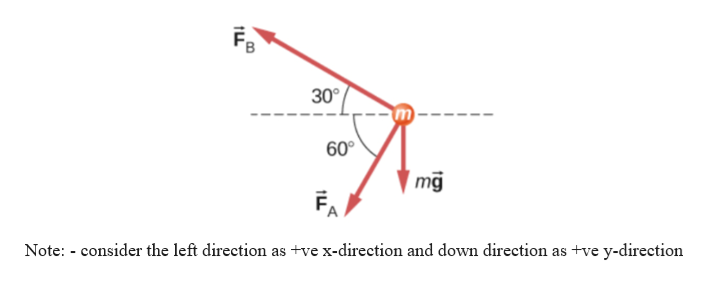Question
3 views

Find the acceleration of the body of mass 10.0 kg
shown below:

check_circle

Step 1

Given information:

Mass of the body (M) = 10 kg

The forces acting on the body:help_outlineImage Transcriptionclose30° 60 mg FA Note: consider the left direction as +ve x-direction and down direction as tve y-direction LL m fullscreen
Step 2

From the diagram the force in the “x direction” are as follows:

Fx = FBcos30 + FAcos60 …… Equation (1)

According to Newton 2nd law the acceleration and net external force can be related as:

F = ma

Hence, we can write the as:

Max = FBcos30 + FAcos60

Step 3

From the diagram the force in the “y direction” are as follows:...

### Want to see the full answer?

See Solution

#### Want to see this answer and more?

Solutions are written by subject experts who are available 24/7. Questions are typically answered within 1 hour.*

See Solution
*Response times may vary by subject and question.
Tagged in

### Newtons Laws of Motion